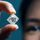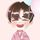cancel
Showing results for
Did you mean:Solution Sage

## What is the key difference between FIRSTNONBLANK and FIRSTNONBLANKVALUE ?

If possible please explain with examples,,,

1 ACCEPTED SOLUTIONCommunity Support

Hi @ddpl ,

FIRSTNONBLANKVALUE() returns the first non-blank value of <expression> corresponding to the sorted values of <column>.

``FIRSTNONBLANKVALUE(<column>, <expression>)``

This function is different from FIRSTNONBLANK in that the <column> is added to the filter context for the evaluation of <expression>. It is equivalent to :

``````=
CALCULATE (
<Expression>,
FIRSTNONBLANK ( <Column Name>, CALCULATE (<Expression> ) )
)
``````

I have built a simlply data sample for you to understand:

``````Measure 1 = FIRSTNONBLANK('Table'[Type],CALCULATE(SUM('Table'[Value])))

Measure 2 = FIRSTNONBLANKVALUE('Table'[Type],CALCULATE(SUM('Table'[Value])))

Measure 3 = FIRSTNONBLANKVALUE('Table'[Type],SUM('Table'[Value]))``````• Measure 1 returns the first Type in the list whose Value are not empty, Since Type is Text ,it will in alphabetical order ,is "B"
• Measure 2 returns the Value corresponding to the first Type whose Value is not empty, that is, the Value of "B"= the result of the Measure 1 , is 2
• Measure 3 is the same as Measure 2

Refer to:

FIRSTNONBLANKVALUE function (DAX) - DAX | Microsoft Docs

Best Regards,
Eyelyn Qin
If this post helps, then please consider Accept it as the solution to help the other members find it more quickly.

2 REPLIES 2Community Support

Hi @ddpl ,

FIRSTNONBLANKVALUE() returns the first non-blank value of <expression> corresponding to the sorted values of <column>.

``FIRSTNONBLANKVALUE(<column>, <expression>)``

This function is different from FIRSTNONBLANK in that the <column> is added to the filter context for the evaluation of <expression>. It is equivalent to :

``````=
CALCULATE (
<Expression>,
FIRSTNONBLANK ( <Column Name>, CALCULATE (<Expression> ) )
)
``````

I have built a simlply data sample for you to understand:

``````Measure 1 = FIRSTNONBLANK('Table'[Type],CALCULATE(SUM('Table'[Value])))

Measure 2 = FIRSTNONBLANKVALUE('Table'[Type],CALCULATE(SUM('Table'[Value])))

Measure 3 = FIRSTNONBLANKVALUE('Table'[Type],SUM('Table'[Value]))``````• Measure 1 returns the first Type in the list whose Value are not empty, Since Type is Text ,it will in alphabetical order ,is "B"
• Measure 2 returns the Value corresponding to the first Type whose Value is not empty, that is, the Value of "B"= the result of the Measure 1 , is 2
• Measure 3 is the same as Measure 2

Refer to:

FIRSTNONBLANKVALUE function (DAX) - DAX | Microsoft Docs

Best Regards,
Eyelyn Qin
If this post helps, then please consider Accept it as the solution to help the other members find it more quickly.Super User

If I took the time to answer your question and I came up with a solution, please mark my post as a solution and /or give kudos freely for the effort 🙂 Thank you!

Proud to be a Super User!Announcements#### Exclusive opportunity for Women!

Join us for a free, hands-on Microsoft workshop led by women trainers for women where you will learn how to build a Dashboard in a Day!#### Power Platform Conference-Power BI and Fabric Sessions

Join us Oct 1 - 6 in Las Vegas for the Microsoft Power Platform Conference.Top Solution Authors
Top Kudoed Authors
Users online (1,783)